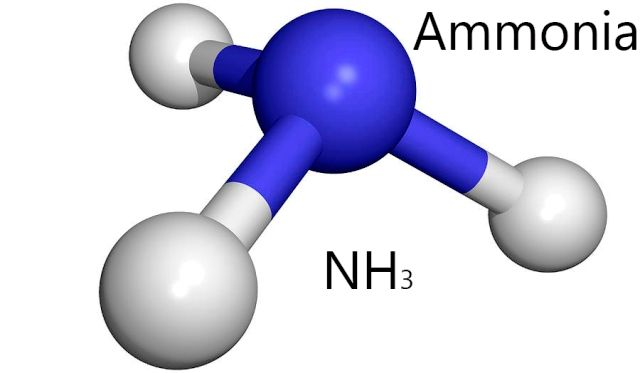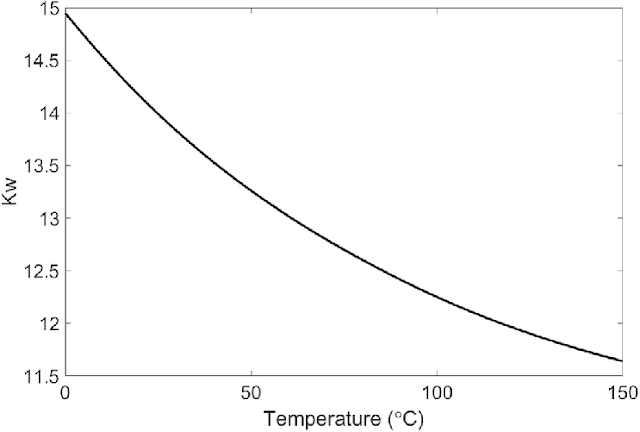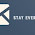### The Lewis Dot Structure for H2OCreated by MakeTheBrainHappy.

This is the Lewis Dot Structure for H2O. You could alternatively also draw the structure by including two dots for every bond. While oxygen's octet seems to have been filled, hydrogen only has two electrons for its valence shell. Based on our previous discussion surrounding the covalent bonds which hydrogen forms, we know that hydrogen's valence shell only requires two electrons because it only has a 1s orbital. Therefore its valence electron is filled by oxygen sharing another electron.

H2O's Lewis Dot Structure gives it many unique properties mostly due to the two lone pairs on the central oxygen atom. This increases electron-electron repulsion and therefore creates a bent structure as opposed to CO2's linear structure. This "bent" molecular structure gives it many unique properties such as being polar. One of the most fascinating phenomena is the idea of "hydrogen bonding" which influences water's properties dramatically.Hydrogen bonding is a type of intermolecular force where the electronegativity difference in the oxygen atom causes a partial permanent dipole to form between the hydrogen and oxygen atoms (shown in the dashed lines). The bonds in the water molecule themselves are covalent bonds. You can learn about the difference in this post. Source

Due to the strength of these hydrogen bonds, water has a relatively high melting and boiling point, although they are not as high as network covalent solids. Those are bonded by intramolecular forces which involve the actual sharing of electrons vs. partial dipole forces in hydrogen bonds. There are only three types of bonds which can hydrogen bond. These are N-H, O-H, and F-H bonds due to the large electronegativity differences between the molecules.NH3 is another molecule which creates similar hydrogen bonds as H2O. Source

How does hydrogen bonding impact the melting and boiling point of water?

Common knowledge indicates that the melting point of water is 0˚C whereas the boiling point is 100˚C. This is due to the exceptional strength of hydrogen bonds as an intermolecular force. Comparing it to the two other "simple" structures which undergo hydrogen bonding; namely, HF (hydrogen fluoride) and NH3 (ammonia), we see that H2O actually has a higher melting and boiling point than either of these two.

The reason for this can be found by examining the lewis dot structure for H2O. When you look at the structure you notice that it has two lone pair electrons and 2 hydrogen bonds. When you visualize the structures for HF and NH3 (you can see the lewis structure of NH3 in this article) you see that they either have three lone pairs and one hydrogen or three hydrogens and one lone pair. This does not optimize the amount of potential hydrogen bonds; whereas H2O's 2:2 ratio does.

Therefore on average H2O is able to form more hydrogen bonds with itself as indicated by the lewis dot structure of H2O, allowing for an overall stronger intermolecular force. This means that more energy is required the break these intermolecular forces to allow for either melting or boiling (phase transitions) and therefore H2O has a higher freezing/boiling point.

What other properties does water have?

Water is one of the only elements to be found in all three states (solid, liquid and gaseous) naturally on earth. Hydrogen bonding causes a crystalline structure which makes it less dense than liquid water. This is why ice floats in the oceans. Water has also a high surface tension and adhesion. Cohesion and adhesion are essential for plants who manipulate these processes to move water from the roots to the leafs to support the growth of the plant. All this is caused by the simple structure of H2O, represented by the Lewis Dot Diagram above.Water molecules in all three states of matter. Source

How does the lewis dot structure for water relate to acids and bases?

As you can see in the lewis dot structure for H2O, water can be broken up into two constituent ions: H+(aq) and OH-(aq). It may also be helpful to mention that H+ ions, i.e. individual protons, do not actually exist naturally within solution (they exist as H3O+ or hydronium ions). However for the purposes of this article we will consider H+(aq) as equivalent to H3O+(aq). These groups are incredibly important in formulating various environments and reacting to different species. So important in fact that they are very prominent in the definitions for acids and bases.

A specific definition including both was the first developed for acids and bases in 1884 by the Swedish chemist Svante Arrhenius. He stated that acids produce H+ ions and bases produce OH- ions. However this theory does not account for molecules that don't contain these ions and rather interact with water to produce them (such as ammonia - NH3). Nevertheless, more expansive theories dealing with these limitations center around these two very important ions.

So to what extent are these ions produced in pure water?

Pure water here is defined as just containing H2O molecules. As mentioned in our previous analysis of the lewis dot structure of H2O, the intermolecular forces governing behavior are very strong. So are the intramolecular bonds within the molecule which are governed by the large electronegativity difference between hydrogen and oxygen (2.20 vs. 3.44, respectively). Given that the electronegativity scale (originally developed by famous twentieth century chemist Linus Pauling) goes up to four this difference is incredibly significant. As you can imagine therefore the amount of ionization is very low: the solubility constant is approximately 1.00 * 10^-14 at 25˚C.

From this solubility constant you can calculate the approximate molar concentration based on the following equation (the autoionization of water):

2H2O(l) => H3O+(aq) + OH-(aq)

Kw = [H3O+(aq)][OH-]

Since the H3O+(aq) and OH-(aq) are produced in a 1:1 ratio we can replace these with x representing the amount of each ion produced.

1.00*10^-14 = [x][x]

x = 1.00*10^-7 M

This means that .00001% of a pure water solution contains H3O+(aq) with another .00001% containing OH-(aq). On the whole however, this is an incredibly small proportion of the total water solution.

How does this process depend on temperature?

As we mentioned before in our discussions of the lewis dot structure for H2O the intermolecular forces between H2O molecules are very strong. These need to be broken in addition to the intramolecular forces. When you increase the temperature you increase the average amount of energy available to break bonds within the environment. Therefore you would expect there to be a higher molar concentration of these ions in the pure water solutions as you increase the temperature.Kw decreasing as temperature increases => increases molar solubility. Source

As you can see in the graph above this trend does bear out since the Kw is decreasing as temperature increase. Since we are talking about concentrations that are very small decimals decreasing how negative the power is is like increase the concentration. You can perform a similar molar solubility calculation as we did above in order to figure out the concentration at any temperature above (estimating the y-value based on the x-value).

Is H2O polar or nonpolar?

Based on our previous discussions surrounding the lewis dot structure for H2O you could take a pretty good guess what the answer to this question is. However if you are interested in learning more about the answer to this question you can check out our article on the polarity of H2O. The article also goes over the phases of water and the concept of water as a "universal solvent", both what is implied by the term and what the limitations are of water as a solvent.

1.Needs to be more accurate

2.Hello There,

First of all my apologies for a cold email, I am sure you get many of these. My name is Artem Volos, Co-Founder of Clutch Prep - we make videos for tons of science and math classes.

I noticed you have an awesome guide https://www.makethebrainhappy.com/2018/01/lewis-dot-structure-for-h2o.html - about The Lewis Dot Structure for H2O.

I’m just reaching out because I thought that some of the content I have been developing could be a fit for your readers: https://www.clutchprep.com/resources/molecular-electron-geometries

Thanks so much and looking forward to hear from you.

All my best,
Artem

3.Very best keep it up

4.5.